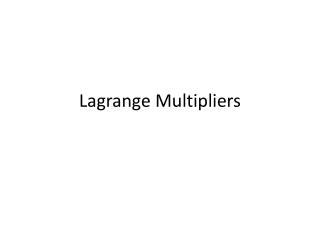DownloadDownload PresentationLagrange Multipliers

Lagrange Multipliers

Télécharger la présentationLagrange Multipliers

- - - - - - - - - - - - - - - - - - - - - - - - - - - E N D - - - - - - - - - - - - - - - - - - - - - - - - - - -
Presentation Transcript

1. Lagrange Multipliers

2. Lagrange Multipliers with One Constraint

3. 1. Use Lagrange multipliers to find the indicated extrema, assuming that x and y are positive(Similar to p.976 #5-10)

4. 2. Use Lagrange multipliers to find the indicated extrema, assuming that x and y are positive(Similar to p.976 #5-10)

5. 3. Use Lagrange multipliers to find the indicated extrema, assuming that x, y and z are positive(Similar to p.976 #11-14)

6. 4. Use Lagrange multipliers to find the indicated extrema of f subject to two constraints. In each case, assume that x, y and z are nonnegative(Similar to p.976 #17-18)

7. 5. Use Lagrange multipliers to find the minimum distance from the curve or surface to the indicated point. [Hint: use min f(x, y) = (x-xo)2 + (y-yo)2(Similar to p.976 #19-25)